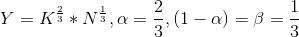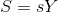### Create an Account

Home / Questions / Assume that the following functions exist and describe production savings investment and c...

# Assume that the following functions exist and describe production savings investment and capital behavior 2 Y KN

Assume that the following functions exist and describe production, savings, investment and capital behavior.a) Solve for output per worker in terms of capital per worker. [Y/L=something in the form of K/L]

b) Assume the government is running a balanced budget, what does this mean for the investment function?

c) Solve for investment in terms of output.

d) How does Capital per Labor change over time? (Give the equation for the Dynamics of Capital and Output). (Keep everything in terms of Capital/Labor ratios). Explain in 1-2 sentences what each term means.

e) Find the Steady State Capital per worker and Output per worker, and Consumption per worker.

f) Now assume the federal government has a surplus. Verbally discuss how this would change your answers above.

2 Y KN,a , (1 a) B ES+T-G SSY Kt+1(1 )Kt

Feb 07 2020 View more View LessGet Solution